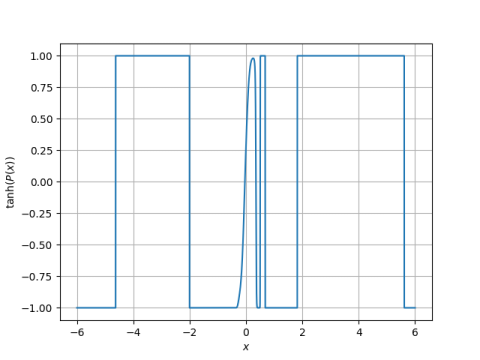# Random polynomials revisited

A few days ago I wrote about the expected number of roots in a random polynomial where each coefficient is drawn from a standard normal, i.e. a Gaussian distribution with mean 0 and variance 1.

Another class of random polynomials, one that comes up in applications to physics, draws each coefficient from a different distribution. Specifically, the nth coefficient in an Nth degree polynomial is drawn from a normal distribution with mean zero and variance N choose n. As before, our reference is .

It turns out that for these random polynomials, the expected number of real zeros is √N. This is not an asymptotic approximation.

For an example, I created a random 44th degree polynomial.

```    from scipy.stats import norm
from scipy.special import binom

N = 44
coeff = [norm.rvs(scale=binom(N,n)**0.5) for n in range(N+1)]
```

I plotted the (tanh of) this polynomial as described here.This polynomial clearly has 8 real roots. The expected number of roots for a 44th degree random polynomial of this type is √44 = 6.63.

 Alan Edelman and Eric Kostlan. How many zeros of a random polynomial are real? Bulletin of the AMS. Volume 32, Number 1, January 1995